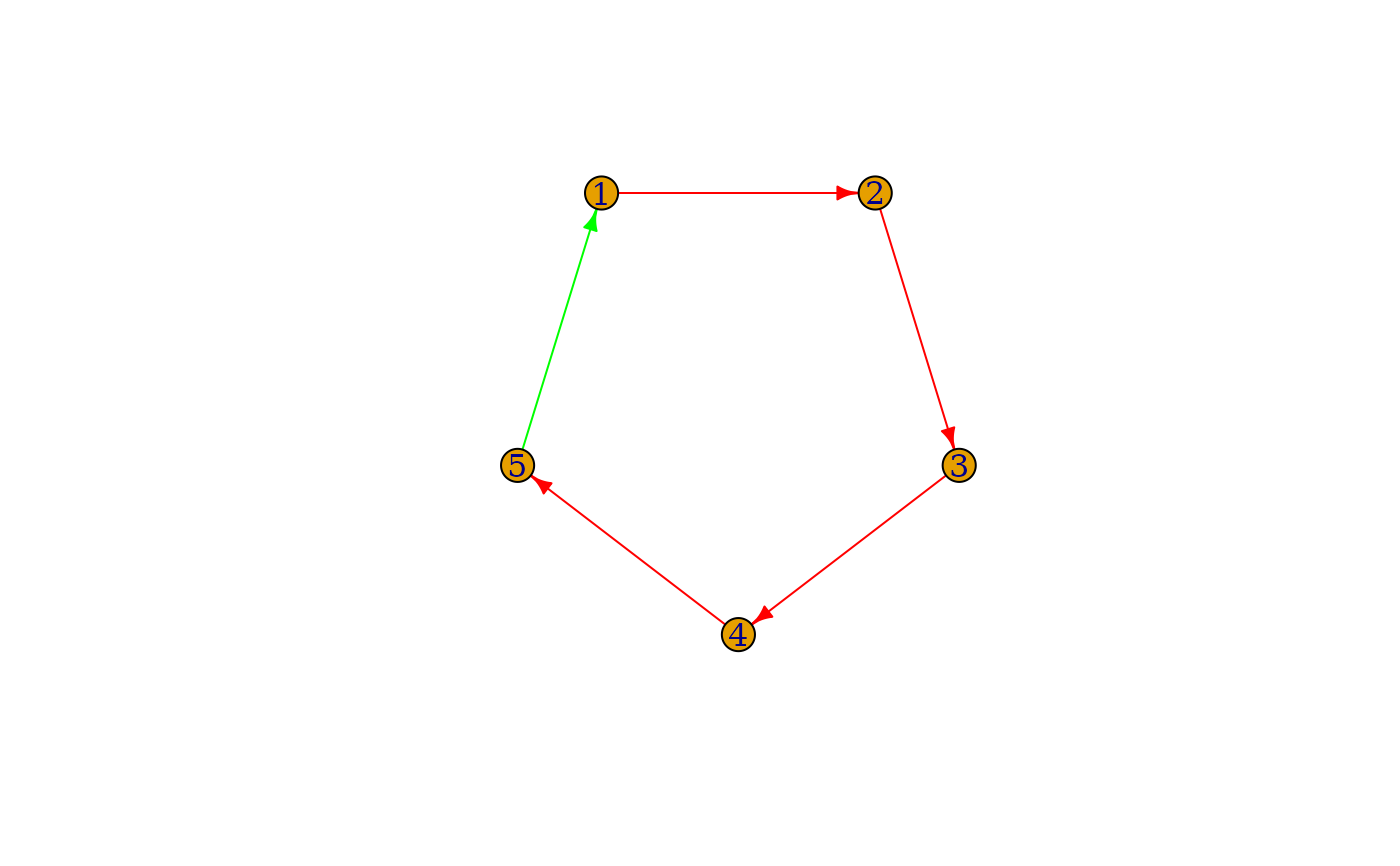The new edges are given as a vertex sequence, e.g. internal numeric vertex ids, or vertex names. The first edge points from edges to edges, the second from edges to edges, etc.

## Usage

add_edges(graph, edges, ..., attr = list())

## Arguments

graph

The input graph

edges

The edges to add, a vertex sequence with even number of vertices.

...

attr

## Value

The graph, with the edges (and attributes) added.

## Details

If attributes are supplied, and they are not present in the graph, their values for the original edges of the graph are set to NA.

Other functions for manipulating graph structure: +.igraph(), add_vertices(), complementer(), compose(), connect(), contract(), delete_edges(), delete_vertices(), difference.igraph(), difference(), disjoint_union(), edge(), igraph-minus, intersection.igraph(), intersection(), path(), permute(), rep.igraph(), reverse_edges(), simplify(), union.igraph(), union(), vertex()

## Examples

g <- make_empty_graph(n = 5) %>%
1, 2,
2, 3,
3, 4,
4, 5
)) %>%
set_edge_attr("color", value = "red") %>%